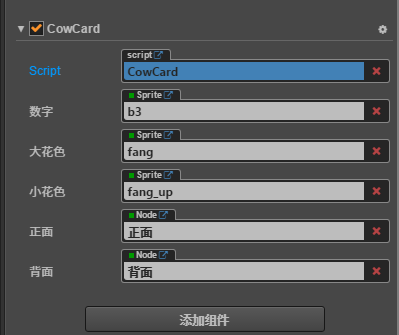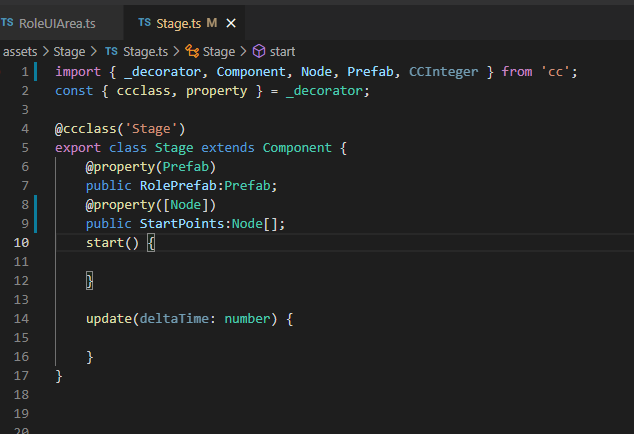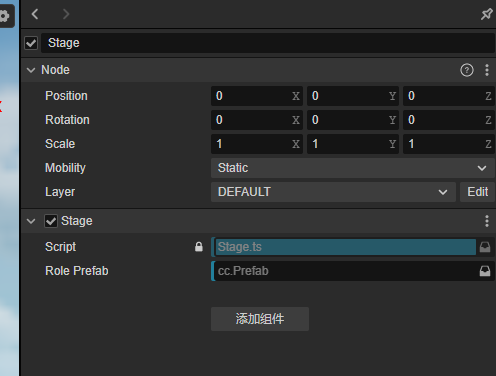# 请问properties里面可以定义对象数组嘛？谢谢

• 当声明属性是一个数组时，可以在声明处填写他们的类型或构造函数来完成声明，如：
``````    properties: {
any: [],      // 不定义具体类型的数组
bools: [cc.Boolean],
strings: [cc.String],
floats: [cc.Float],
ints: [cc.Integer],

values: [cc.Vec2],
nodes: [cc.Node],
frames: [cc.SpriteFrame],
}
``````
• 或者
``````properties: {
names: {
default: [],
type: [cc.String]   // 用 type 指定数组的每个元素都是字符串类型
},

enemies: {
default: [],
type: [cc.Node]     // type 同样写成数组，提高代码可读性
},
}
``````
3赞

``````var MyClass = cc.Class(...);
....
properties: {
names: {
default: [],
type: [MyClass]
}
}
.....
``````
2赞

1.4.2版本出现数组不能序列化的问题1.5也有类似问题

1赞

@property([Node])
public StartPoints:Node[];

@property(Array)
public StartPoints:Array;@property(Node)

``````startPoints:Node[] = [];
``````

@property(Node)
public StartPoints:number;

``````@property({
type: [Node]
})
startPoints: Node[] = [];
``````
``````@property([Node])
startPoints:Node[] = [];
``````